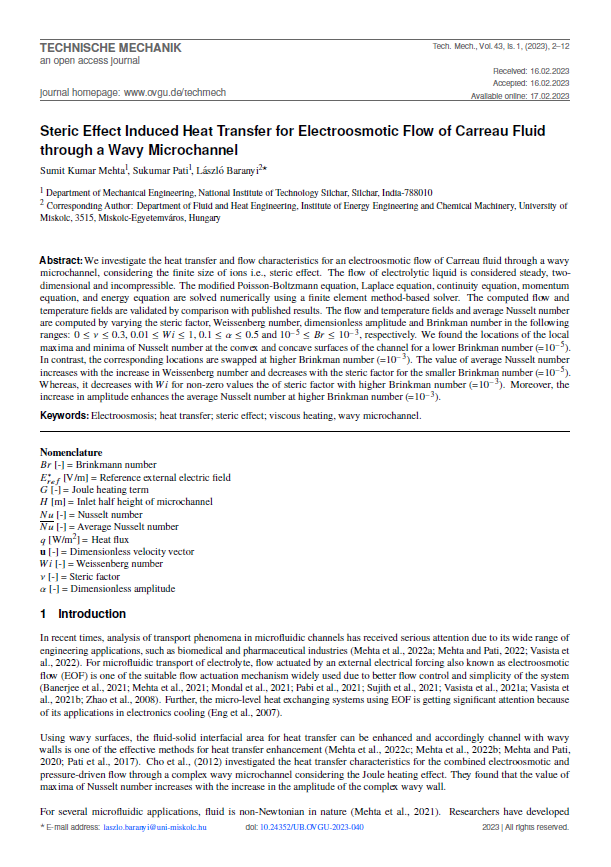# Steric Effect Induced Heat Transfer for Electroosmotic Flow of Carreau Fluid through a Wavy Microchannel

## Authors

• Sumit Kumar Mehta IIT Guwahati
• Sukumar Pati NIT Silchar
• László Baranyi Department of Fluid and Heat Engineering, Institute of Energy Engineering and Chemical Machinery, University of Miskolc

## Keywords:

Electroosmosis, heat transfer, steric effect, viscous heating, wavy microchannel

## Abstract

We investigate the heat transfer and flow characteristics for an electroosmotic flow of Carreau fluid through a wavy microchannel, considering the finite size of ions i.e., steric effect. The flow of electrolytic liquid is considered steady, two-dimensional and incompressible. The modified Poisson-Boltzmann equation, Laplace equation, continuity equation, momentum equation, and energy equation are solved numerically using a finite element method-based solver. The computed flow and temperature fields are validated by comparison with published results. The flow and temperature fields and average Nusselt number are computed by varying the steric factor, Weissenberg number, dimensionless amplitude and Brinkman number in the following ranges: 0≤ υ ≤0.3, 0.01≤Wi ≤1, 0.1≤ α ≤ 0.5 and 10-5≤ Br ≤10-3, respectively. We found the locations of the local maxima and minima of Nusselt number at the convex and concave surfaces of the channel for a lower Brinkman number (=10-5). In contrast, the corresponding locations are swapped at higher Brinkman number (=10-3). The value of average Nusselt number increases with the increase in Weissenberg number and decreases with the steric factor for the smaller Brinkman number (=10-5). Whereas, it decreases with Wi for non-zero values the of steric factor with higher Brinkman number (=10-3). Moreover, the increase in amplitude enhances the average Nusselt number at higher Brinkman number (=10-3).2023-02-16

## How to Cite

Mehta, S. K., Pati, S., & Baranyi, L. (2023). Steric Effect Induced Heat Transfer for Electroosmotic Flow of Carreau Fluid through a Wavy Microchannel. Technische Mechanik - European Journal of Engineering Mechanics, 43(1), 2–12. https://doi.org/10.24352/UB.OVGU-2023-040

Article